# 【剑指Offer】56、数组中出现的次数

2021/8/21 10:52:43 浏览：

# 题目简介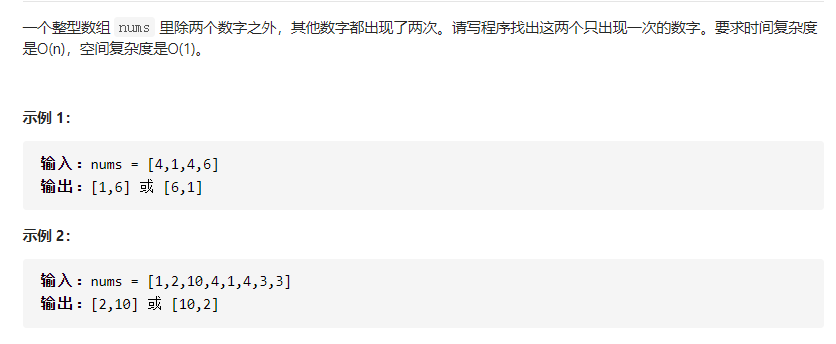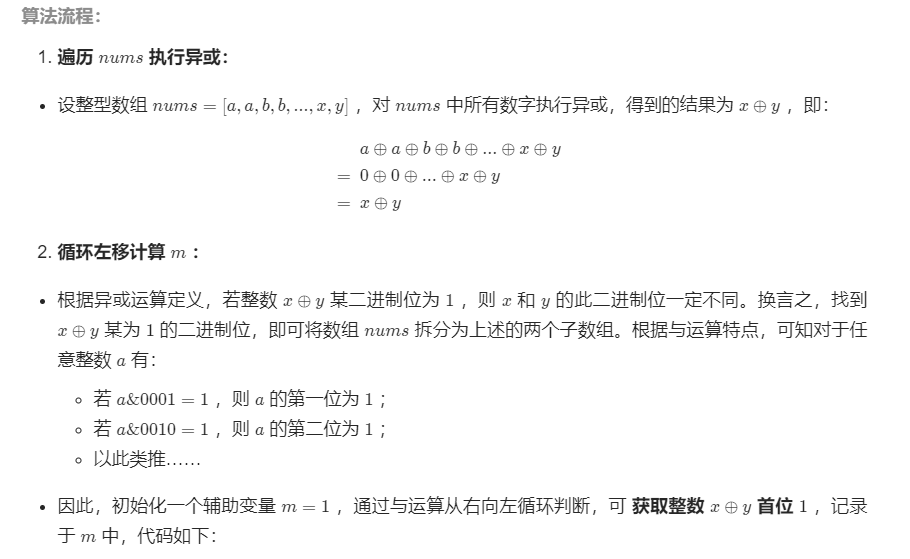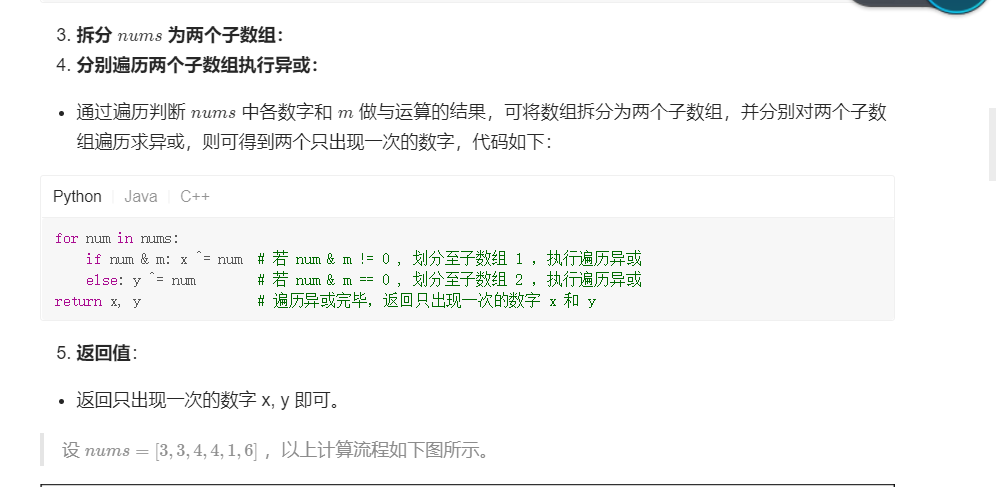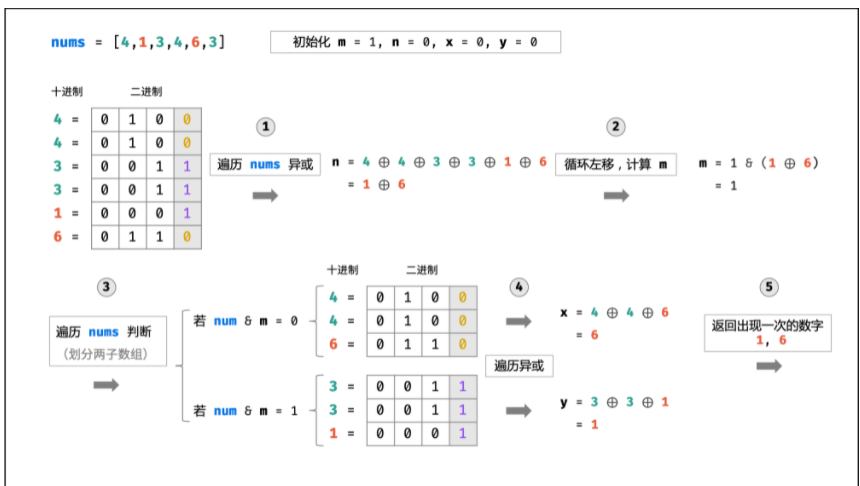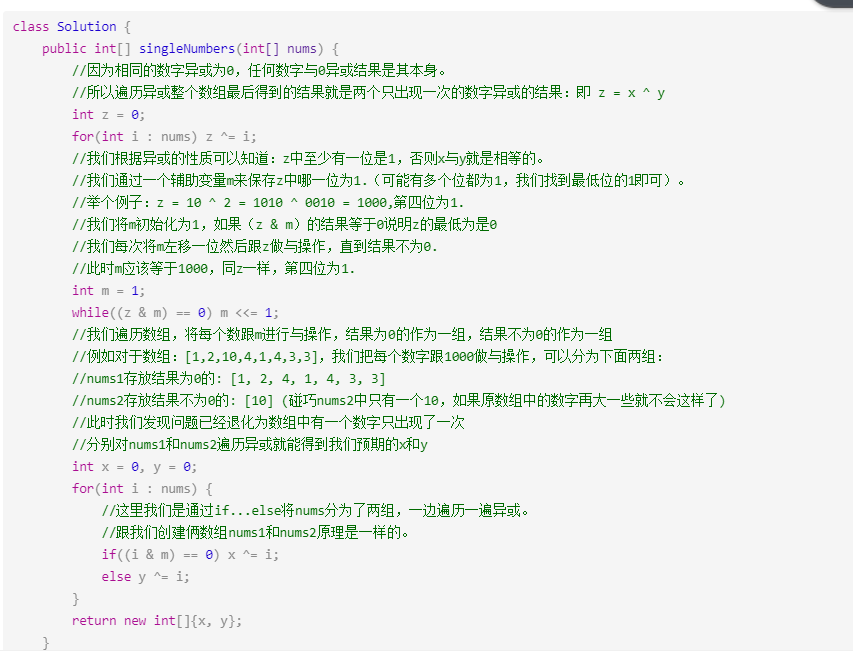``````class Solution {
public int[] singleNumbers(int[] nums) {
int x = 0,y = 0,n = 0,m = 1;
for(int num : nums){
n ^= num;
}

while((m & n) == 0){
m <<= 1;
}

for(int num : nums){
if((num & m) == 0){
x ^= num;
}else{
y ^= num;
}
}
return new int[]{x,y};
}
}
``````